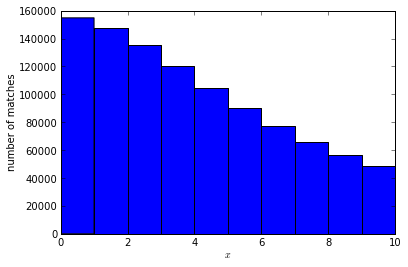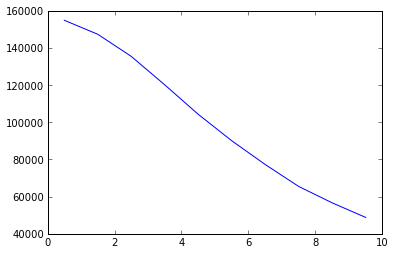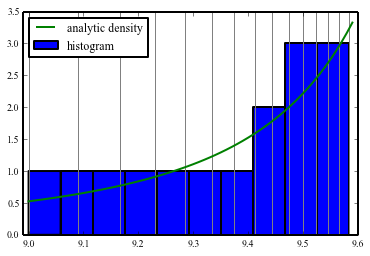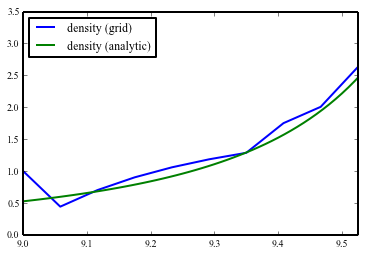# Coding: python particle to grid interpolation

[latexpage]
In particle tracking simulations you often need to interpolate particles onto a grid in one or more dimensions. Recently I decided to write a linear particle-to-grid interpolation in one dimension in python.This is an educational introduction into interpolating particles onto a one-dimensional grid.

## Interpolation of particles onto a grid

Particles are usually described by a vector of coordinates in an $n$-dimensional phase space. Often people want to compute a density of particles along one of the coordinate axes. Let us first start with the example of particles in a two-dimensional space, they have coordinates $(x,x’)$. A common question will be: What is the density of the particles projected on the $x$ axis?

note: code blocks from here on are executed one after another in an ipython notebook. You can download the notebook here.

To answer the question you first have to think about: How do you compute the density? A builtin way in python/pylab is the histogram function.

%pylab inline
x=10*tan(random.random(1000000))/tan(1)
# Generate 1 Million particles
figure()
hist(x,bins=10) # Calculate the histogram
xlabel("x")
ylabel("number of matches")
show()The histogram function computes the number of particles between the left and right edges of the bars respectively. We can also look at the data:

numbers,bins=histogram(x,bins=10)
print "there are %d particles with x between\
%0.2f and %0.2f" % (numbers, bins,bins)


there are 154841 particles with x between 0.00 and 0.1 

The histogram function does exactly this, count the number of particles between the edges of a bin. Visually the edges are represented by filling a bar between the left and the right edge up to a height proportional to the resulting number of particles.

Another way to look at this would be to say: the histogram function computes the density at the centers of the bins by attributing each particle to its nearest neighbouring bin. We could then compute a value for the density as follows:

numbers,bins=histogram(x,bins=10)
# compute the width of a bin
binwidth=bins-bins
# compute the positions of the centers of the bins
bincenters=(bins[1:]+bins[0:-1])/2
# plot the density (particles per length unit)
plot(bincenters,numbers/binwidth)This looks nice and smooth, but you might say. But if you think about it, for a particle in the middle of two bins, say at the right edge of the first bin at 0.95, is it really justified to assume that it only contributes to the grid point at 0.5? It virtually has the same distance to the grid point at 1.5!
$\begin{figure}\begin{center} \begin{tikzpicture} \fill [red] (0.95,.15) circle (3pt); \draw [line width=2pt] (0,0) — (11,0); \foreach \x in {0,…,11} {\draw [line width=2pt] (\x,0)–(\x,0.3); } \foreach \x in {0,…,10} { \node () at ((\x,-.3) + (0.5,0)) {\x}; } %\fill [red] (0.95,.15) circle (2pt); \end{tikzpicture}\end{center} \caption{A grid with one particle at 0.95} \end{figure}$

This problem can become even worse. Let us define point positions $$x_i=10(1-1/i)$$. Here see a figure with particles with $i$ between 10 and 25:

$\begin{figure}\begin{center} \begin{tikzpicture} \foreach \x in {10,…,25}{ \pgfmathparse{(10*(1-1.0/\x)-9)/.05833} \fill [red] (\pgfmathresult,.15) circle (3pt); } \foreach \x in {0,1,…,10} { \node () at ((\x,-.3) + (0.5,0)) {\x}; } \draw [line width=2pt] (0,0) — (11,0); \foreach \x in {0,…,11} {\draw [line width=2pt] (\x,0)–(\x,0.3); } \end{tikzpicture}\end{center} \caption{A grid with particles of decreasing spacing} \end{figure}$

You can see how the particle distance (which is inversely proportional to the intuitive density) changes smoothly. The density per bin can be calculated analytically to be $\rho(x)=\frac{x \delta}{(-10 + x)^2}$ with $\delta$ the bin width. Let us plot the point positions and the resulting histogram for $i$ between 10 and 25. The histogram however has a step-shape as you can see below and does not compare too well to the analytic result (grey vertical lines indicate particle positions, the green curve is the analytic particle density):

x=10*(1-1/numpy.arange(10,25.)) # the points
density=lambda x: (10/(10/(-10 + x) + (10/(-10 + x))**2))**(-1)*.05833
for i in x:
axvline(i,color="grey",linewidth="1") # grey lines for the point positions
hist(x,bins=10) # histogram of point positions
plot(numpy.arange(9,9.6,.01),density(numpy.arange(9,9.6,.01))) # analytic density function
xlim(8.99,9.6)You see how steppy the histogram looks when you compare it to the analytically calculated density? Maybe we can do better. If we think about the single particle from above again
$\begin{figure}\begin{center} \begin{tikzpicture} \fill [red] (0.95,.15) circle (3pt); \draw [line width=2pt] (0,0) — (11,0); \foreach \x in {0,…,11} {\draw [line width=2pt] (\x,0)–(\x,0.3); } \foreach \x in {0,…,10} { \node () at ((\x,-.3) + (0.5,0)) {\x}; } %\fill [red] (0.95,.15) circle (2pt); \end{tikzpicture}\end{center} \caption{A grid with one particle at 0.95} \end{figure}$
how about we say: It should contribute to its neighbouring bins according to its distance to the bin center. There are the two bin centers at $x_1=0.5$ and $x_2=1.5$ neighbouring the particle. We should pay attention that the total amount of density created by the particle stays the same. Let us for the time being focus on the assumption that a particle only contributes to two bins. So let us zoom in on the particle and its two neighbouring bins:
$\begin{figure}\begin{center} \begin{tikzpicture} \fill [red] (2.85,.15) circle (7pt); \draw [line width=2pt] (0,0) — (6,0); \foreach \x in {0,3,6} {\draw [line width=2pt] (\x,0)–(\x,0.3); } \foreach \x in {0,3} { \draw [line width=2pt] ((\x,0) + (1.5,0)) –((\x,-.3) + (1.5,0)); } \node () at ((0.5,-.7) + (1.5,0)) {0.5\delta}; \node () at ((3,-.7) + (1.5,0)) {1.5\delta}; %\fill [red] (0.95,.15) circle (2pt); \draw[line width=2pt,||] (2.85,.5) — (4.5,.5) node[above,pos=0.5]{0.55\delta}; \draw[line width=2pt,||] (1.5,.5) — (2.85,.5) node[above,pos=0.5]{0.45\delta}; \end{tikzpicture}\end{center} \caption{A grid with one particle at 0.95} \end{figure}$
The easiest way to distribute the particle density between its neighbouring bins is now to just measure its distance $d_i$ to its neighbouring bins, in the example $d_1=0.45\delta,d_2=1-\frac{d_1}{\delta}=0.55\delta$ and add $1-d_i$ to the grid point $i$. This way we make sure that the particle number is conserved (the total number added to the grid is 1, as for the histogram). In our example, the particle contributes $\rho_1=.55, \rho_2=.45$ to the grid bins 1 and 2.

Now expressing this in python and adding the bin width to calculate a proper density we write a function pics2gridpy(particles, left, right, bins). The function is called with four arguments:

particles: the Array of coordinates
left: The left edge of the grid (also the zeroth grid point)
right: The right edge of the grid (no grid point here)
bins: the number of bins (including the one at the left edge)

def pics2gridpy(particles, left, right, bins):
grid=numpy.zeros(bins,dtype=numpy.float64)
binwidth=1.*(right-left)/bins
invbinwidth=1/binwidth
leftIndexF = 0.
binPosition= 0.
for i in range(particles.shape):
leftIndex=particles[i]
(leftIndexF,binPosition)=divmod((particles[i]-left),binwidth)
binPosition*=invbinwidth
leftIndex=int(leftIndexF)
grid[leftIndex % bins]+=1-binPosition
grid[(leftIndex + 1) % bins]+=binPosition
return numpy.array([numpy.arange(left,right,binwidth),grid])


Let’s try to run it on our example particles and again compare to the analytic density!

# because actually the method makes a circular grid, we need to add one bin
# to the left and right of the distribution
bins,density=pics2gridpy(x,min(x)-.05833333,max(x)+0.05833333,12)
plot(bins,density,label="density (grid)")
plot(numpy.arange(9,9.6,.01),density(numpy.arange(9,9.6,.01)),label="density (analytic)")
xlim(min(x),max(x)-0.05833)
pylab.legend(loc="upper left")We see, this is much better. But one thing is notable: The spike for the bin at 9.0, where does it originate? In this region, the density of perticles per bin is smaller than one. Because of that you have to expect aliasing effects that can produce these spikes. In fact, normally you try to have at least a few tens of particles in the bins that matter to you because you want to have a good approximation of the real density. In our case we needed very few because we had a smooth distribution. In most cases however you sum random particles and therefore you expect some noisiness due to the random fluctuations of your distribution.

Now that we have the method let’s get an estimate of its performance for very many particles:

x=random.random(100000)
%timeit bins,density=pics2gridpy(x,0,1,100)


gives a time of 844 msec on my machine. The histogram however only takes 8.5msec. There has to be a way of optimizing this. Next post we will look at how fast we can make it using cython!